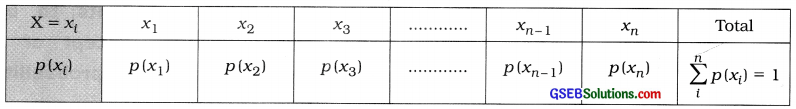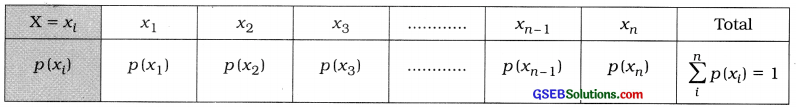# GSEB Class 12 Statistics Notes Part 2 Chapter 2 Random Variable and Discrete Probability Distribution

This GSEB Class 12 Commerce Statistics Notes Part 2 Chapter 2 Random Variable and Discrete Probability Distribution Posting covers all the important topics and concepts as mentioned in the chapter.

## Random Variable and Discrete Probability Distribution Class 12 GSEB Notes

Random Variable:
Let U be a sample space of a random experiment. A function which associates a real number with each outcome of U, is called a random variable and is denoted by symbol X. Symbolically, it is also represented by X: U → R.
1. Discrete Random Variable:
A random variable X, which is capable of assuming all the values in a set of real numbers or its subsets, is called a discrete random variable. Examples of a discrete random variable are: number of children per family, getting number of heads when a coin is tossed three times.

2. Continuous Random Variable:
If a random variable X is an element of R or its subset, whose interval is (a, b), where a < b and is capable of assuming any value in the interval, then that random variable X is called a continuous random variable. Examples of continuous random variable are: age of a person, temperature of a place, production of a company.

Discrete Probability Distribution:
Suppose, probability of a discrete random variable X is P[X = xi] = p(xi). If for i = 1, 2, 3, …, n; p(xi) > 0 and $$\sum_{i=1}^{n} p\left(x_{i}\right)$$ = 1, then set of real values {p(x1), p(x2), …. p(xn)} is called probability distribution of a discrete random variable X.

In tabular form, it is written as under:Here, 0 < p(xi) < 1 and i = 1, 2, 3, …, nMean and Variance of a Discrete Random Variable:
Suppose X is a discrete random variable that assumes any one value from x1, x2, ……… xn and the probability distribution of X is as follows:Here, 0 < p(xi) < 1 and i = 1, 2, 3, …, n
Mean (p) and variance (σ2) are also called the mean and variance of probability distribution of X.

Binomial Probability Distribution:
Law of Dichotomy:
If possible outcomes of an experiment are only two, the rule is called law of dichotomy.

• Success: If outcome of an experiment is certain, it is called success.
• Failure: If outcome of an experiment is not certain, it is called failure.

Dichotomous Experiment:
An experiment, which has only two outcomes, namely Success or Failure, is called a dichotomous experiment.

Bernoulli Trials:
If a dichotomous experiment is repeated n times and in each trial probability of success (S) p(0 < p < 1) is constant, then all such trials are called Bernoulli trials.

Binomial Random Variable:
If in the series of successes (S) and failures (F) obtained in n numbers of Bernoulli trials, the number of successes (S) is denoted by X, then X is called binomial random variable. X is capable of taking any value of a finite set {0, 1, 2, …, n}.

Binomial Probability Distribution:
If probability distribution of x, a value of a binomial random variable X is P(X = x) = p(x) = nCx pxqn-x
Where, n = Number of Bernoulli trials; x = 0, 1, 2, …, n; 0 <p < 1 and p + q = 1, then it is called binomial probability distribution.

• n and p are parameters of binomial probability distribution.
• Mean of binomial probability distribution = np and Variance of binomial probability distribution = npq
• If the probability of x successes in N trials of a random experiment is p(x), then the expected frequency of x successes is = N – p(x).

Properties of Binomial Distribution;

• The mean np of the binomial distribution shows the expected number (average) of successes in n Bernoulli trials.
• The variance of the distribution is = npq. So its standard deviation is = $$\sqrt{n p q}$$
• In the distribution np > npq.
• Probability of failure q = $$\frac{n p q}{n p}$$
• If p < $$\frac{1}{2}$$, skewness of the distribution is positive.
• If p > $$\frac{1}{2}$$, skewness of the distribution is
negative.
• If p = $$\frac{1}{2}$$, skewness of the distribution is zero and it becomes symmetrical distribution.

Symbols:

• X = Random variable
• x = Value of random variable X
• P (X = x) = Probability of x, a value of X
• n = Number of Bernoulli trials
• x = Number of successes
• p = Probability of success
• q = Probability of failure = 1 -pFormulae:
1. Mean of Random Variable X:
μ = E(X) = Σx . p(x)

2. Variance of Random Variable X:
σ2 = V(X) = E(X – μ)2
= E (X2) – [E(X)]2
= Σx2p(x)- [Σx – p(x)]2

3. Binomial Probability Distribution:
P(X = x) = p(x) = nCx px qn-x Where, n = Number of Bernoulli trials; x = 0, 1, 2 …… n
p – Probability of success (0 <p < 1)
q = 1 – p